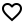## assertk

Project Url: willowtreeapps/assertkIntroduction: assertions for kotlin inspired by assertj
More: Author   ReportBugs
Tags:

assertions for kotlin inspired by assertj

## Setup

``````repositories {
mavenCentral()
}

dependencies {
testCompile 'com.willowtreeapps.assertk:assertk-jvm:0.23'
}
``````

### Javascript/Common

Replace dependency on `assertk-jvm` with `assertk-js` or `assertk` to use it in JavaScript and common projects, respectively.

## Usage

Simple usage is to wrap the value or property you are testing in `assertThat()` and call assertion methods on the result.

``````import assertk.assertThat
import assertk.assertions.*

class PersonTest {
val person = Person(name = "Bob", age = 18)

@Test
fun testName() {
assertThat(person.name).isEqualTo("Alice")
// -> expected:<["Alice"]> but was:<["Bob"]>
}

@Test
fun testAge() {
assertThat(person.age, "age").isGreaterThan(20)
// -> expected [age] to be greater than:<20> but was:<18>
}

@Test
fun testNameProperty() {
assertThat(person::name).isEqualTo("Alice")
// -> expected [name]:<["Alice"]> but was:<["Bob"]>
}
}
``````

You can see all built-in assertions in the docs.

### Nullability

Since null is a first-class concept in kotlin's type system, you need to be explicit in your assertions.

``````val nullString: String? = null
assertThat(nullString).hasLength(4)
``````

will not compile, since `hasLength()` only makes sense on non-null values. You can chain `isNotNull()` to handle this.

``````val nullString: String? = null
assertThat(nullString).isNotNull().hasLength(4)
// -> expected to not be null
``````

This will first ensure the string is not null before running any other checks.

### Multiple assertions

You can assert multiple things on a single value by providing a lambda as the second argument. All assertions will be run even if the first one fails.

``````val string = "Test"
assertThat(string).all {
startsWith("L")
hasLength(3)
}
// -> The following 2 assertions failed:
//    - expected to have length:<3> but was:<"Test"> (4)
``````

You can wrap multiple assertions in an `assertAll` to ensure all of them get run, not just the first one.

``````assertAll {
assertThat(false).isTrue()
assertThat(true).isFalse()
}
// -> The following 2 assertions failed:
//    - expected to be true
//    - expected to be false
``````

### Extracting data

There's a few ways you extract the data you want to assert on. While you can do this yourself before calling the assertion, these methods will add the extra context to the failure message which can be helpful.

The simplest way is with `prop()`. It will take a property (or a name and a lambda) and return an assertion on that property.

``````val person = Person(age = 22)
assertThat(person).prop(Person::age).isEqualTo(20)

// -> expected [age]:<2> but was:<2> (Person(age=22))
``````

For collections, you can use `index()` to pull a specific index from a list, and `key()` to pull a specific value from a map.

``````assertThat(listOf(1, 2, 3)).index(1).isEqualTo(1)

// -> expected: []:<1> but was:<2> ([1, 2, 3])

assertThat(mapOf("one" to 1, "two" to 2, "three" to 3)).key("two").isEqualTo(1)

// -> expected: [["two"]]:<1> but was:<2> ({"one"=1, "two"=2, "three"=3})
``````

You can also extract a property from a collection using `extracting()`.

``````val people = listOf(Person(name = "Sue"), Person(name = "Bob"))
assertThat(people)
.extracting(Person::name)
.containsExactly("Sue", "Bob")
``````

### Exceptions

If you expect an exception to be thrown, you can use the version of `assertThat` that takes a lambda.

``````assertThat {
throw Exception("error")
}.isFailure().hasMessage("wrong")
// -> expected [message] to be:<["wrong"]> but was:<["error"]>
``````

This method also allows you to assert on successfully returned values.

``````assertThat { 1 + 1 }.isSuccess().isNegative()
// -> expected to be negative but was:<2>
``````

### Table Assertions

If you have multiple sets of values you want to test with, you can create a table assertion.

``````tableOf("a", "b", "result")
.row(0, 0, 1)
.row(1, 2, 4)
.forAll { a, b, result ->
assertThat(a + b).isEqualTo(result)
}
// -> the following 2 assertions failed:
//    on row:(a=<0>,b=<0>,result=<1>)
//    - expected:<> but was:<>
//    on row:(a=<1>,b=<2>,result=<4>)
//    - expected:<> but was:<>
``````

Up to 4 columns are supported.

## Custom Assertions

One of the goals of this library is to make custom assertions easy to make. All assertions are just extension methods.

``````fun Assert<Person>.hasAge(expected: Int) {
prop(Person::age).isEqualTo(expected)
}

assertThat(person).hasAge(20)
// -> expected [age]:<2> but was:<2> (Person(age=22))
``````

For completely custom assertions, you have a few building blocks. `given` will give you the actual value to assert on, and `expected()` and `show()` will help you format your failure message.

``````fun Assert<Person>.hasAge(expected: Int) = given { actual ->
if (actual.age == expected) return
expected("age:\${show(expected)} but was age:\${show(actual.age)}")
}

assertThat(person).hasAge(20)
// -> expected age:<20> but was age:<22>
``````

You can also build assertions that chain by using `transform`. This allows you to both assert on the actual value, and return something more specific that additional assertions can be chained on.

``````fun Assert<Person>.hasMiddleName(): Assert<String> = transform(appendName("middleName", seperator = ".")) { actual ->
if (actual.middleName != null) {
actual.middleName
} else {
expected("to not be null")
}
}

assertThat(person).hasMiddleName().isEqualTo("Lorie")

// -> expected [middleName]:to not be null
``````

Note: this is a bit of a contrived example as you'd probably want to build this out of existing assertions instead.

``````fun Assert<Person>.hasMiddleName(): Assert<String> = prop(Person::middleName).isNotNull()
``````

The general rule of thumb is to prefer building out of the existing assertions unless you can give a more meaningful error message.

## Contributing to assertk

Contributions are more than welcome! Please see the Contributing Guidelines and be mindful of our Code of Conduct.

Apps